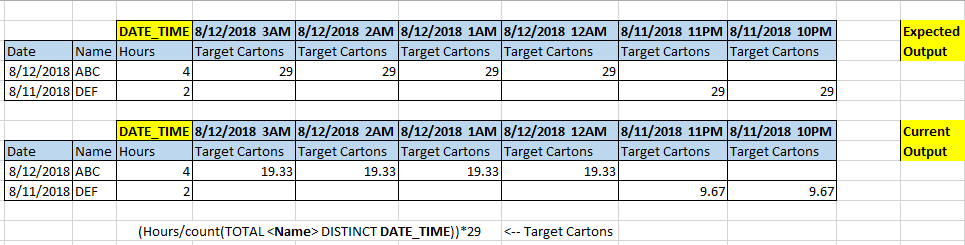New to QlikView

Discussion board where members can get started with QlikView.

Highlighted
Contributor II

Count Total Distinct Help

Hi Guys,

Please correct me in the below formula.

Target Cartons = (Hours/count(TOTAL <Name> DISTINCT DATE_TIME))*29

I am trying to distribute the hours worked for each hour and calculate target based on it. If ABC worked 4 hours, it should consider only 8/12 date and DATE_TIME of 8/12 and distribute among it and multiply by 29, so 1*29 = 29. But with above formula, it also counts the DATE_TIME of previous day and hence gives the output in the below second table.

How do I correct the formula so as to get only the count of current days hours?Tags (3)
1 Solution

Accepted Solutions
Contributor II

Re: Count Total Distinct Help

I think I got it, changed from Name to Date

(Hours/count(TOTAL <Date> DISTINCT DATE_TIME))*29

Thank you for your responses though Vishwarath.

4 Replies
Esteemed Contributor III

Re: Count Total Distinct Help

May be add Date field like

Target Cartons = (Hours/count(TOTAL <Name>  {<Date = {[\$(=Date(DATE_TIME, 'M/DD/YYYY')))]} >} DISTINCT DATE_TIME))*29

Contributor II

Re: Count Total Distinct Help

No change in output, gives the same result.

Esteemed Contributor III